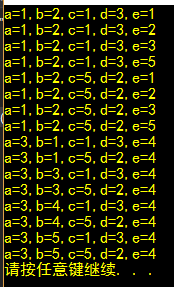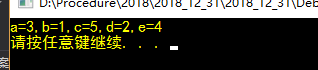# C【练习】（逻辑题）

### （1） 5位运动员参加了10米台跳水比赛，有人让他们预测比赛结果

#### E选手说：我第四，A第一；

1.我自己先提取转化成数字，因为计算机只接受数字的逻辑，汉子的逻辑它看不懂
A: B2，A3；
B: B2 , E4;
C: C1 , D4;
D: C5 , D3;
E：E4 , A1;

1.我们首先把所有的条件遍历出来 for循环5层嵌套从A>>>E
2,条件的确认，既然题目中怎么说我们就怎么将其用计算机语言表达出来
if (((b == 2) + (a == 3) == 1)
&& ((b == 2) + (e == 4) == 1)
&& ((c == 1) + (d == 2) == 1)
&& ((c == 5) + (d == 3) == 1)
&& ((e == 4) + (a == 1) == 1))

–3.这点我要加亮标记，int a = 0, b = 0, c = 0, d = 0, e = 0;

for (a = 1; a < 5; a++){
for (b = 1; b <= 5; b++){
for (c = 1; c <= 5; c++){
for (d = 1; d <= 5; d++){
for (e = 1; e <= 5; e++){
if (((b == 2) + (a == 3) == 1)
&& ((b == 2) + (e == 4) == 1)
&& ((c == 1) + (d == 2) == 1)
&& ((c == 5) + (d == 3) == 1)
&& ((e == 4) + (a == 1) == 1)){
if ((a*b*c*d*e) == 120){
printf("a=%d,b=%d,c=%d,d=%d,e=%d\n", a, b, c, d, e);
}

}
}
}
}
}
}
}


#### 已知3个人说了真话，1个人说的是假话。

/*每种情况 有两种 不是0/1*/
int a, b, c, d;
for (a = 0; a <= 1; a++){
for (b = 0; b <= 1; b++){
for (c = 0; c <= 1; c++){
for (d = 0; d <= 1; d++){
if (((a == 0) +
(c == 1) +
(d == 1) +
(d != 1)) == 3){
if ((a + b + c + d) == 1){
printf("a=%d,b=%d,c=%d,d=%d\n", a, b, c, d);
}
}
}
}
}

}


int kil_1(){
char kil;
for (kil = 'A'; kil < 'D'; kil++){
if ((kil != 'A') +
(kil == 'C') +
(kil == 'D') +
(kil != 'D') == 3){
printf("%c", kil);
}
}
}